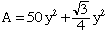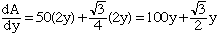Quandaries and Queries Subject: maxima problem Name: evelina Who is asking: Student Level: Secondary Question: Hi, I'm having troubles with taking the derrivative in this maxima problem. Here's the actual question and my work so far... A window is the shape of a rectangle with an equilateral triangle on top. The perimeter of the window is 300 cm. Find the width that will let the maximum light to enter. ``` /\ let y be the length of the y / \ y sides of the triangle and /____\ width of the rectangle in cm | y | let x be the length of the | | rectangle in cm x| | x |_____| y ``` Maximize: Area= (area of rectangle) + (area of triangle) ``` 3^(1/2) = xy + ------ y^2 *1* 4 ``` Given: Perimeter= 300cm 2x + 3y = 300 x = 50y    *2* Sub *2* into *1* ``` 3^(1/2) A= 50y^2 + ------- y^2 4 ``` For maximum, ``` dA ---- = 0 dy dA ---- = 100y + ???? dy ``` This is where my problem is. I cannot figure out how to take the derrivative of the square root of 3 etc. I've come up with many different answers but I know none of them work, when I set the derivative equal to zero I always get y to equal zero. Did I set up the problem incorrectly or is my problem just not knowing how to take the derrivative? Thanks so much for your time, Evelina Hi Evelina, The square root of 3 divided by 4 is a constant just like 50 is a constant so ifthenThe rreason that this answer "doesn't work" is that there is an error in your equation *2*. Since the perimeter is 300 cm you have correctly that 2x + 3y = 300 however, solving for y you should get 3y = 300 - 2x and hence y = 100 - 2/3 x       *2* Substitite this equation *2* into *1* and differentiate. Cheers, Penny Go to Math Central